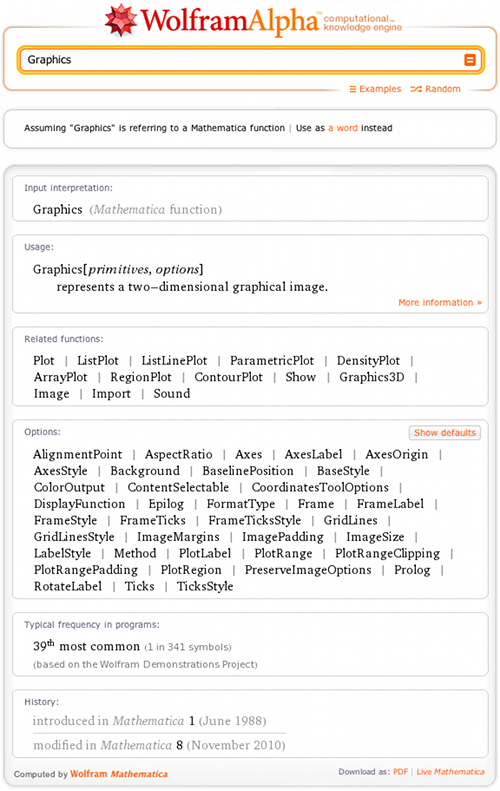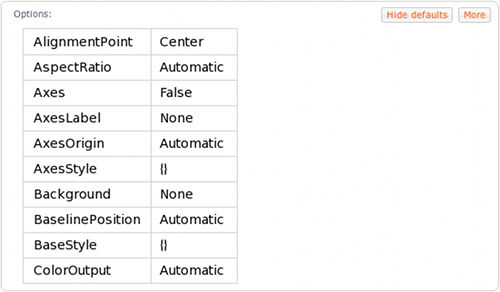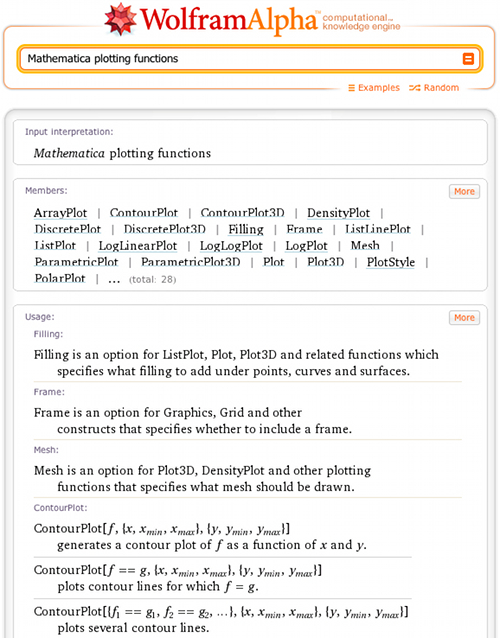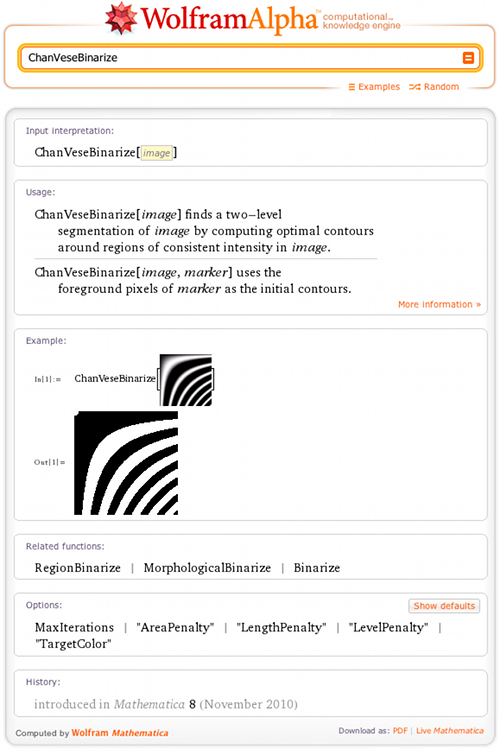# Making Mathematica Computable

October 3, 2011 —Comments Off

As most Wolfram|Alpha blog readers know, the engine behind the Wolfram|Alpha computational knowledge engine is Wolfram Research’s powerful mathematics and computation software, Mathematica. Ironically, while Wolfram|Alpha contains thousands of datasets on diverse and sundry subject areas, until very recently, its computable knowledge of the Mathematica language itself has been somewhat limited.

No longer! Wolfram|Alpha now not only knows about all core Mathematica functions, but it can also provide a fair bit of computational information about them. For example, take the useful Mathematica function FoldList:As you can see, Wolfram|Alpha now gives the usage syntax and a list of related functions (all of which are hyperlinked for additional exploration), as well as usage frequency and revision history information for this function (and many others).

As another example, take the case of the Mathematica wrapper function Graphics:We again get useful information about the function, but since this function takes options, we also see a list of them. Furthermore, clicking “Show defaults” then reveals the default values corresponding to these options:You can ask for more specific information, such as “Mathematica Pi revision history”:Try querying classes of functions in certain usage areas, such as “Mathematica plotting functions”:Or obtain a listing of Mathematica functions with certain characteristics, such as “Mathematica functions having the Constant attribute”:A selected but growing number of functions also show usage examples, like, for instance, ChanVeseBinarize:More functionality is on the way, including additional visualizations, such as the neighborhood graph of related functions, illustrated below for the function FoldList:In summary, Wolfram|Alpha now provides a convenient, computational way to explore the Mathematica language that complements its already extensive online documentation. We hope you (and especially those of you who are also Mathematica users) find these new features both useful and interesting.

Cool! I’ve been wanting something like this for a long time. I really like the Neighborhood graph idea. Waiting to see if I can find the top 10 most used functions.

Posted by Seth Chandler October 3, 2011 at 2:17 pm

Very good. So Wolfram Alpha is a subset of Mathematica. Please can you define which features of Mathematica are excluded from Wolfram Alpha.

Posted by Brian Gilbert October 5, 2011 at 1:31 am

Eric, I really appreciated your tutorial (Foldlist,graphics… It is one of the best items I have seen on the blog. Would sure like to see tutorials like this regularly.

Posted by Warren Heller October 5, 2011 at 12:29 pm

That’s absolutely amazing, I am so happy 😀

Posted by George October 12, 2011 at 8:59 am

Wonderful! But unfortunately the pre processing of it behaves strange. Try to type following expressions:

Take[ {1,2,3,4},2]
Drop[ {1,2,3,4},2]
Select[ Range[1,10], PrimeQ]
Select[ Range[1,10], EvenQ]
Count[ Range[1,10], a_/; PrimeQ[a] ]
Total[ {1,2,3} ]

I have more strange examples.

Posted by yuko matsuda December 19, 2011 at 12:33 am

How can I write the function (x+y)/(x^2+y^2) in mathematica and get the same results like by Wolfram Alpha

Posted by Taha January 1, 2012 at 10:03 am